# 那个深秋的夜晚——与ADC的浪漫邂逅

## ADC简介

STM32f103 系列有 3 个 ADC，精度为 12 位，每个 ADC 最多有 16 个外部通道，可测量16个外部和2个内部信号源。其中ADC1 和 ADC2 都有 16 个外部通道，ADC3 根据 CPU 引脚的不同通道数也不同，一般都有8 个外部通道。

ADC的结果可以左对齐或右对齐方式存储在16位数据寄存器中。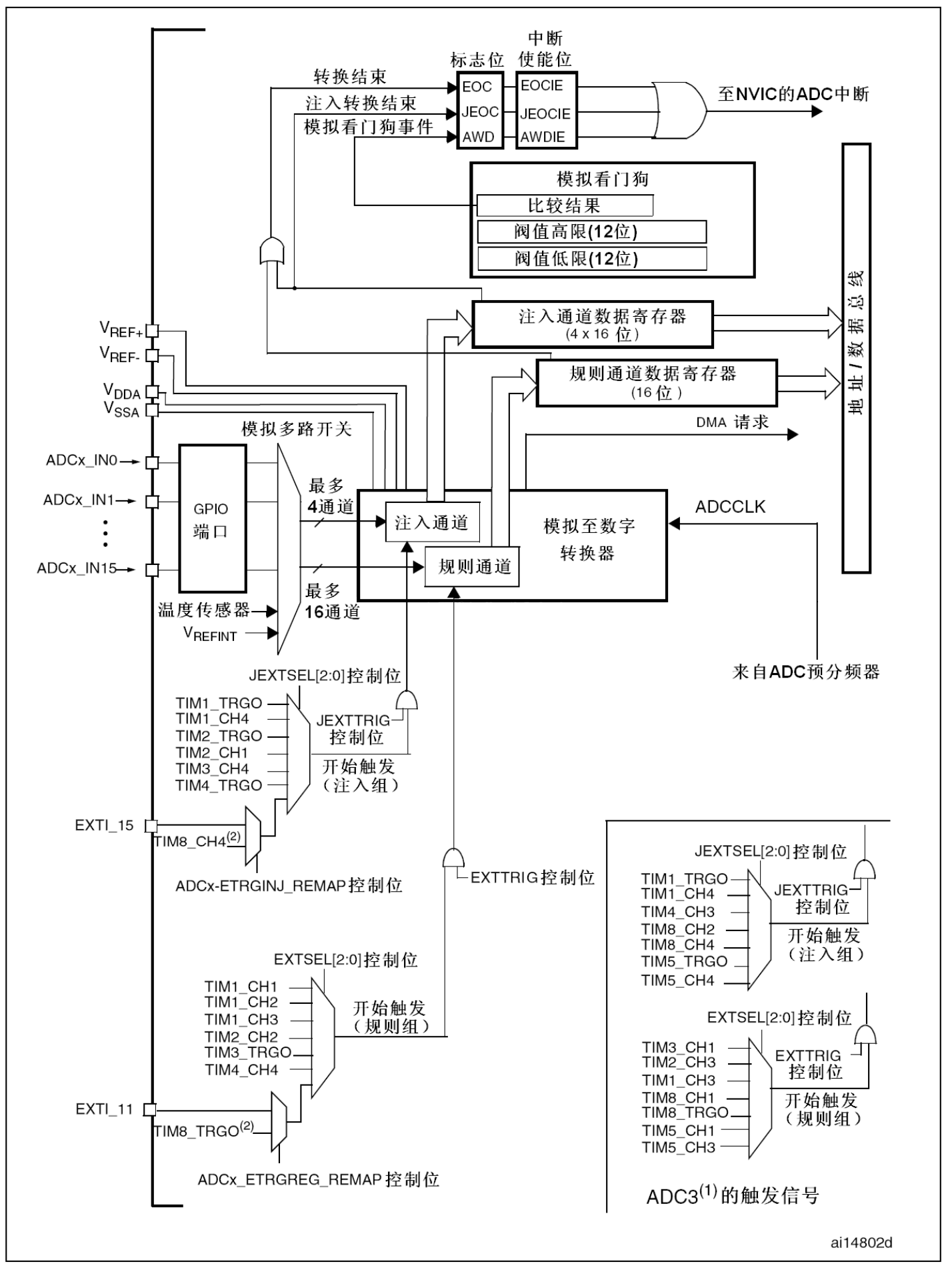### 电压输入范围

VDD： D即device 表示器件的意思，是器件内部的工作电压。

VDDA(A表示 Analog 【模拟】)是模拟电源，当使用到模拟信号的时候，比如AD（模数）或者DA（数模）的时候，系统会使用VDDA的电压作为参考电压来。不要求精准使用的话，可以直接把VDDA和VDD同时接入$3.3V$就行。如果要求精准，则需要做一个稳压电路，再接入VDDA 。

VSS：S即series 表示公共连接的意思，通常指电路公共接地端电压 ，VSSA同理为模拟部分的电源。

#### 定制电压范围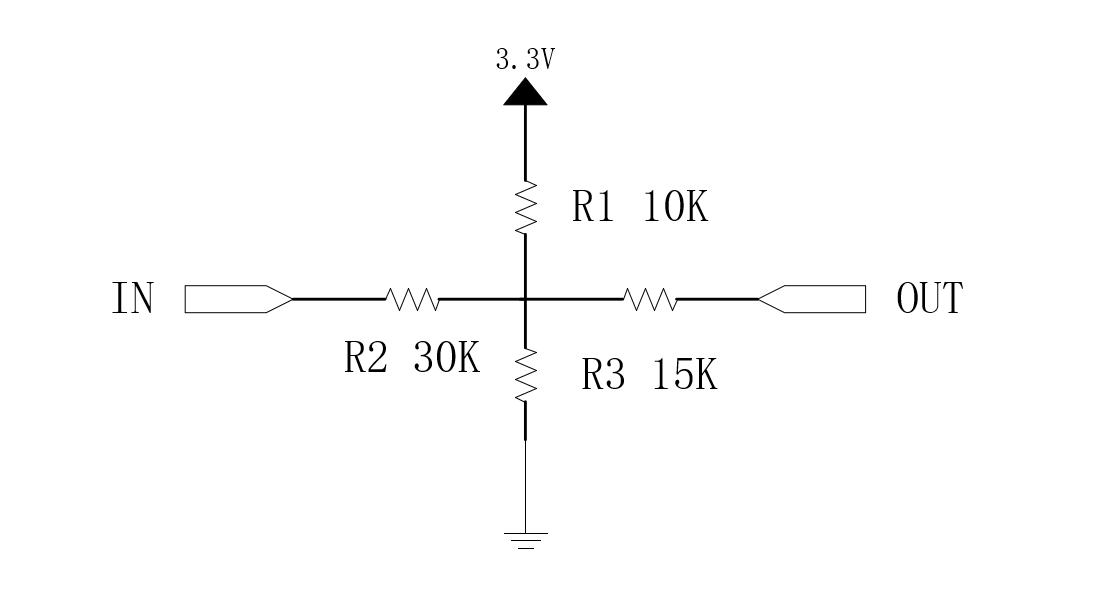$${(V_{IN}-V_{OUT})\over 2 } + {(3.3V-V_{OUT})\over R_1 } = {V_{OUT} \over R_3}$$

### 输入通道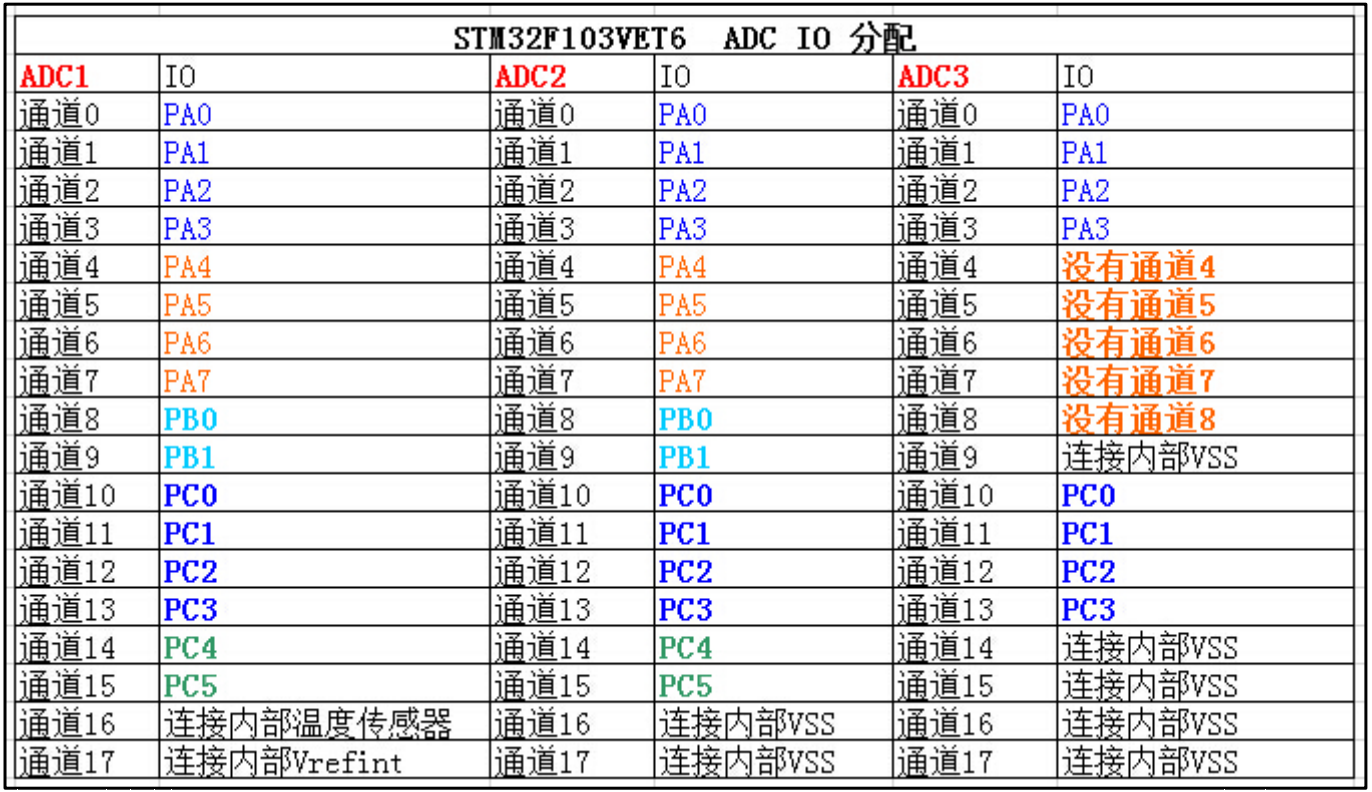• 规则通道：也就是一般情况下用到的通道；
• 注入通道：可以在规则通道转换的时候强行插入转换，享有转换特权，有点像中断的操作。

### 转换顺序

• 规则序列

转换的优先级并不是根据通道的编号来确定的，需要操作规则序列寄存器SQRx(x=1,2,3)，例如将通道六设置为第十个转换，SQ10这个位写6即可。SQL1中SQL位用于设置转换通道的数量，具体的操作都可以库函数代劳。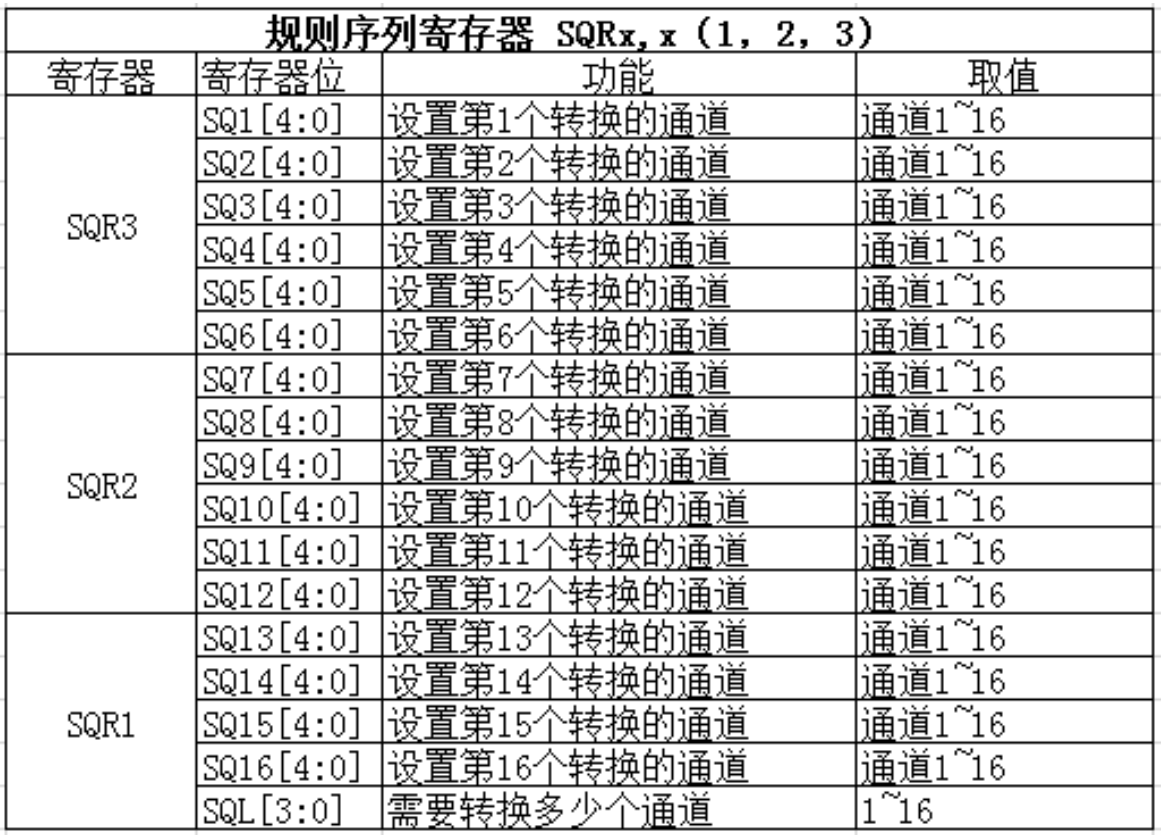• 注入序列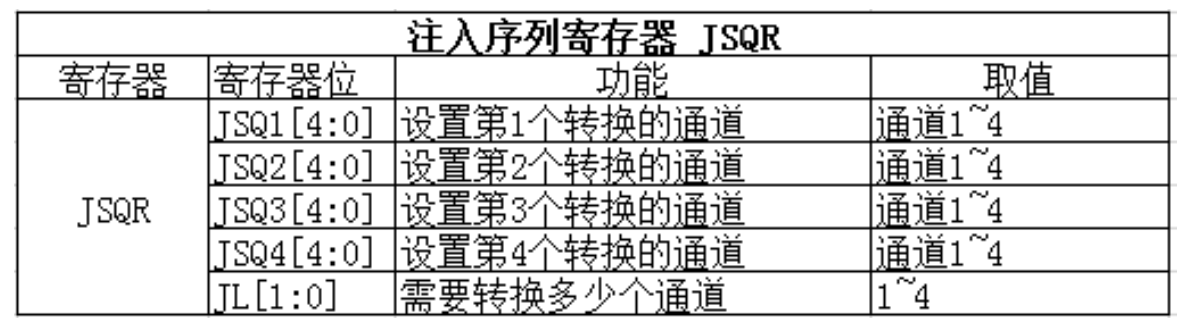注入序列的转换顺序稍有不一样，当JL的值小于4时，第一次转的顺序是JSQx(x=4-JL)；当JL等于4时，与SQR一致。

### 触发源

• 软件触发

ADC_CR2ADON位写1，开始转换；

• 外部事件触发

外部触发源很多，不在此详叙，主要经过EXTSEL[2:0]JEXTSEL[2:0]位来选择，EXTTRIGJEXTTRIG这两位来激活。

### 转换时间

#### ADC时钟

ADC 输入时钟 ADC_CLK 由 PCLK2 经过分频产生，最大是 14M，分频因子由 RCC 时钟配置寄存器RCC_CFGR 的位 15:14 ADCPRE[1:0]设置，可以是 2/4/6/8 分频，注意这里没有 1 分频。一般我们设置 $PCLK2=HCLK=72M$。

#### 采样时间

ADC 使用若干个 ADC_CLK 周期对输入的电压进行采样，采样的周期数可通过 ADC 采样时间寄存器 ADC_SMPR1ADC_SMPR2 中的 SMP[2:0]位设置，ADC_SMPR2 控制的是通道 09，ADC_SMPR1控制的是通道 1017。每个通道可以分别用不同的时间采样。其中采样周期最小是 1.5 个，即如果我们要达到最快的采样，那么应该设置采样周期为 1.5个周期，这里说的周期就是$1\over ADC_{CLK}$。

ADC 的转换时间跟 ADC 的输入时钟和采样时间有关，公式为：
$$Tconv = 采样时间 + 12.5 个周期。$$

### 数据寄存器

ADC转换后，规则组数据存放在ADC_DR寄存器中，注入组在JDRx

#### 规则数据寄存器

ADC 规则组数据寄存器 ADC_DR 只有一个，是一个 32 位的寄存器，低 16 位在单 ADC时使用，高 16 位是在 ADC1 中双模式下保存 ADC2 转换的规则数据，双模式就是 ADC1 和ADC2 同时使用。在单模式下，ADC1/2/3 都不使用高 16 位。因为 ADC 的精度是 12 位，

#### 注入数据寄存器

ADC 注入组最多有 4 个通道，刚好注入数据寄存器也有 4 个，每个通道对应着自己的寄存器，不会跟规则寄存器那样产生数据覆盖的问题。ADC_JDRx 是 32 位的，低 16 位有效，高 16 位保留，数据同样分为左对齐和右对齐，具体是以哪一种方式存放，由
ADC_CR2 的 11 位ALIGN 设置。

### 中断

#### 转换结束中断

• 规则通道转换结束中断，对应中断位：ADC_IT_EOC;

• 注入转换通道转换结束中断，对应中断位：ADC_IT_JEOC;

• 模拟看门狗中断，对应中断位：ADC_IT_AWD

### 双 ADC 模式

AD 转换包括采样阶段和转换阶段，在采样阶段才对通道数据进行采集；而在转换阶段只是将采集到的数据进行转换为数字量输出，此刻通道数据变化不会改变转换结果。独立模式的 ADC 采集需要在一个通道采集并且转换完成后才会进行下一个通道的采集。而双重 ADC 的机制就是使用两个 ADC 同时采样一个或者多个通道。双重 ADC 模式较独立模式一个最大的优势就是提高了采样率，弥补了单个 ADC 采样不够快的缺点。

## 例程设计

STM32 的 ADC 功能繁多，以下通过三个基础例程尽量展示ADC的功能，代码中均没有使用宏定义，是为了看得更直观。

### 独立模式单通道

*1) *初始 ADC 用到的 GPIO，这里我们以GPIOC1为例：

void ADCx_GPIO_Config(void){
GPIO_InitTypeDef GPIO_InitStructure;

// 打开 ADC IO 端口时钟
RCC_APB2PeriphClockCmd(RCC_APB2Periph_GPIOC, ENABLE);

// 配置 ADC IO 引脚模式
// 必须为模拟输入
GPIO_InitStructure.GPIO_Pin = GPIO_Pin_1;
GPIO_InitStructure.GPIO_Mode = GPIO_Mode_AIN;
// 初始化 ADC IO
GPIO_Init(GPIOC, &GPIO_InitStructure);
}

2) 设置 ADC 的工作参数并初始化（以ADC1为例）；

void ADCx_Mode_Config(void){
ADC_InitTypeDef ADC_InitStructure;
// 打开 ADC 时钟
RCC_APB2PeriphClockCmd(RCC_APB2Periph_ADC1, ENABLE);
// ADC 模式配置
// 只使用一个 ADC，属于独立模式
ADC_InitStructure.ADC_Mode = ADC_Mode_Independent;

// 禁止扫描模式，多通道才要，单通道不需要
ADC_InitStructure.ADC_ScanConvMode = DISABLE;

// 连续转换模式
ADC_InitStructure.ADC_ContinuousConvMode = ENABLE;

// 不用外部触发转换，软件开启即可
ADC_InitStructure.ADC_ExternalTrigConv = ADC_ExternalTrigConv_None;

// 转换结果右对齐
ADC_InitStructure.ADC_DataAlign = ADC_DataAlign_Right;

// 转换通道 1 个
ADC_InitStructure.ADC_NbrOfChannel = 1;
// 初始化 ADC
ADC_Init(ADC1, &ADC_InitStructure);
// 配置ADC时钟为PCLK2的8分频，即9MHz
RCC_ADCCLKConfig(RCC_PCLK2_Div8);

// 配置 ADC 通道转换顺序和采样时间
ADC_RegularChannelConfig(ADC1, ADC_Channel_13, 1, ADC_SampleTime_55Cycles5);
/**
* @param1 ADC 外设
* @param2 通道选择
* @param3 转换顺序 1~16
* @param4 采样周期
*/
// ADC 转换结束产生中断，在中断服务程序中读取转换值
ADC_ITConfig(ADC1, ADC_IT_EOC, ENABLE);

// 开启ADC，并开始转换
ADC_Cmd(ADC1, ENABLE);

// 初始化ADC 校准寄存器
ADC_ResetCalibration(ADC1);
// 等待校准寄存器初始化完成
while(ADC_GetResetCalibrationStatus(ADC1));

// ADC开始校准
ADC_StartCalibration(ADC1);
// 等待校准完成
while(ADC_GetCalibrationStatus(ADC1));

// 由于没有采用外部触发，所以使用软件触发ADC转换
ADC_SoftwareStartConvCmd(ADC1, ENABLE);
}

*3) *配置中断源和中断优先级；

void ADC_NVIC_Config(void){
NVIC_InitTypeDef NVIC_InitStructure;
// 优先级分组
NVIC_PriorityGroupConfig(NVIC_PriorityGroup_1);

// 配置中断优先级
NVIC_InitStructure.NVIC_IRQChannel = ADC1_2_IRQn;
NVIC_InitStructure.NVIC_IRQChannelPreemptionPriority = 1;
NVIC_InitStructure.NVIC_IRQChannelSubPriority = 1;
NVIC_InitStructure.NVIC_IRQChannelCmd = ENABLE;
NVIC_Init(&NVIC_InitStructure);
}

4) 中断服务函数

void ADC1_2_IRQHandler(void){
if (ADC_GetITStatus(ADC1,ADC_IT_EOC)==SET) {
// 读取ADC的转换值
ADC_ConvertedValue = ADC_GetConversionValue(ADC1);
// ADC_ConvertedValue为转换值，定义原型为：__IO uint16_t ADC_ConvertedValue;
}
ADC_ClearITPendingBit(ADC1,ADC_IT_EOC);
}

5) main()函数

extern __IO uint16_t ADC_ConvertedValue;
// 局部变量，用于保存转换计算后的电压值
float ADC_ConvertedValueLocal;

int main(void){
// 默认已经完成了串口配置
USART_Config();
ADCx_GPIO_Config();
ADCx_Mode_Config();
ADC_NVIC_Config();
printf("\t\n ----这是一个串口发送实验----\r\n");
ADC_ConvertedValueLocal =(float) ADC_ConvertedValue/4096*3.3;
printf("\r\n The current AD value = 0x%04X \r\n",ADC_ConvertedValue);
printf("\r\n The current AD value = %f V \r\n",ADC_ConvertedValueLocal);
printf("\r\n\r\n");
}

### 独立模式多通道

*1) *初始 ADC 用到的 GPIO；

GPIO_InitStructure.GPIO_Pin =     ADC_PIN1 | ADC_PIN2 | ADC_PIN3 | ADC_PIN4 | ADC_PIN5 | ADC_PIN6;

2) 设置 ADC 的工作参数并初始化，还需要对DMA进行配置；

    DMA_InitTypeDef DMA_InitStructure;
RCC_AHBPeriphClockCmd(RCC_AHBPeriph_DMA1, ENABLE);                    // 打开 DMA 时钟
DMA_InitStructure.DMA_PeripheralBaseAddr = (u32)(&(ADC_x->DR));        // 外设基址为：ADC 数据寄存器地址
DMA_InitStructure.DMA_MemoryBaseAddr = (u32)ADC_ConvertedValue;        // 存储器地址,用户自定义变量（数组名）
DMA_InitStructure.DMA_DIR = DMA_DIR_PeripheralSRC;                    // 数据源来自外设
DMA_InitStructure.DMA_BufferSize = 6;                                // 缓冲区大小，应该等于数据目的地的数目大小
DMA_InitStructure.DMA_PeripheralInc = DMA_PeripheralInc_Disable;    // 外设寄存器只有一个，地址不用递增
DMA_InitStructure.DMA_MemoryInc = DMA_MemoryInc_Enable;             // 存储器地址递增
// 外设数据大小为半字，即两个字节
DMA_InitStructure.DMA_PeripheralDataSize = DMA_PeripheralDataSize_HalfWord;
DMA_InitStructure.DMA_MemoryDataSize = DMA_MemoryDataSize_HalfWord; // 内存数据大小也为半字，跟外设数据大小相同
DMA_InitStructure.DMA_Mode = DMA_Mode_Circular;                        // 循环传输模式

// DMA 传输通道优先级为高，当使用一个DMA通道时，优先级设置不影响
DMA_InitStructure.DMA_Priority = DMA_Priority_High;

// 禁止存储器到存储器模式，因为是从外设到存储器
DMA_InitStructure.DMA_M2M = DMA_M2M_Disable;

DMA_Init(DMA1_Channel1, &DMA_InitStructure);                        // 初始化DMA
DMA_Cmd(DMA1_Channel1 , ENABLE);                                    // 使能 DMA 通道
/* -------------------------------------------------------------------------------------------------------*/

ADC_InitStructure.ADC_ScanConvMode = ENABLE;                        // 扫描模式 多通道
ADC_InitStructure.ADC_NbrOfChannel = 6;                                // 转换通道个数

// 配置ADC 通道的转换顺序和采样时间
ADC_RegularChannelConfig(ADC1, ADC_Channel_10, 1, ADC_SampleTime_55Cycles5);
ADC_RegularChannelConfig(ADC1, ADC_Channel_11, 2, ADC_SampleTime_55Cycles5);
ADC_RegularChannelConfig(ADC1, ADC_Channel_12, 3, ADC_SampleTime_55Cycles5);
ADC_RegularChannelConfig(ADC1, ADC_Channel_13, 4, ADC_SampleTime_55Cycles5);
ADC_RegularChannelConfig(ADC1, ADC_Channel_14, 5, ADC_SampleTime_55Cycles5);
ADC_RegularChannelConfig(ADC1, ADC_Channel_15, 6, ADC_SampleTime_55Cycles5);

ADC_DMACmd(ADC1, ENABLE);                                            // 使能ADC DMA 请求

3) main()函数

// ADC1转换的电压值通过DMA方式传到SRAM
extern __IO uint16_t ADC_ConvertedValue;

// 局部变量，用于保存转换计算后的电压值
float ADC_ConvertedValueLocal;

int main(void){
// 默认已经完成了串口配置
USART_Config();
ADCx_GPIO_Config();
ADCx_Mode_Config();;
printf("\t\n ----这是一个串口发送实验----\r\n");
ADC_ConvertedValueLocal =(float) ADC_ConvertedValue/4096*3.3;
ADC_ConvertedValueLocal =(float) ADC_ConvertedValue/4096*3.3;
ADC_ConvertedValueLocal =(float) ADC_ConvertedValue/4096*3.3;
ADC_ConvertedValueLocal =(float) ADC_ConvertedValue/4096*3.3;
ADC_ConvertedValueLocal =(float) ADC_ConvertedValue/4096*3.3;
ADC_ConvertedValueLocal =(float) ADC_ConvertedValue/4096*3.3;

printf("\r\n CH0 value = %f V \r\n",ADC_ConvertedValueLocal);
printf("\r\n CH1 value = %f V \r\n",ADC_ConvertedValueLocal);
printf("\r\n CH2 value = %f V \r\n",ADC_ConvertedValueLocal);
printf("\r\n CH3 value = %f V \r\n",ADC_ConvertedValueLocal);
printf("\r\n CH4 value = %f V \r\n",ADC_ConvertedValueLocal);
printf("\r\n CH5 value = %f V \r\n",ADC_ConvertedValueLocal);
printf("\r\n\r\n");
}

### 双ADC同步规则

*1) *初始 ADC 用到的 GPIO；

    GPIO_InitStructure.GPIO_Pin = GPIO_Pin_1;
GPIO_InitStructure.GPIO_Pin = GPIO_Pin_4;

2) 设置 ADC 的工作参数并初始化，还需要对DMA进行配置；

    ADC_InitStructure.ADC_Mode = ADC_Mode_RegSimult;            // 双ADC的规则同步
ADC_InitStructure.ADC_ScanConvMode = ENABLE ;                 // 扫描模式
ADC_InitStructure.ADC_ContinuousConvMode = ENABLE;            // 连续转换模式
// 不用外部触发转换，软件开启即可
ADC_InitStructure.ADC_ExternalTrigConv = ADC_ExternalTrigConv_None;

ADC_InitStructure.ADC_DataAlign = ADC_DataAlign_Right;        // 转换结果右对齐
ADC_InitStructure.ADC_NbrOfChannel = 1;                           // 转换通道个数
ADC_Init(ADCx_1, &ADC_InitStructure);                        // 初始化ADC
RCC_ADCCLKConfig(RCC_PCLK2_Div8);                             // 配置ADC时钟PCLK2的8分频，即9MHz
// 配置ADC1 通道的转换顺序和采样时间
ADC_RegularChannelConfig(ADC1, ADC_Channel_11, 1, ADC_SampleTime_239Cycles5);
ADC_DMACmd(ADC1, ENABLE);                                    // 使能ADC DMA 请求
// 由于没有采用外部触发，所以使用软件触发ADC转换
ADC_SoftwareStartConvCmd(ADCx_1, ENABLE);

ADC_Init(ADC2, &ADC_InitStructure);
// 配置ADC2 通道的转换顺序和采样时间
ADC_RegularChannelConfig(ADC2, ADC_Channel_14, 1, ADC_SampleTime_239Cycles5);

ADC_ExternalTrigConvCmd(ADC2, ENABLE);                        // 使能ADC2的外部触发转换

3) main()函数

// ADC1转换的电压值通过MDA方式传到SRAM
extern __IO uint32_t ADC_ConvertedValue;

// 局部变量，用于保存转换计算后的电压值
float ADC_ConvertedValueLocal[NOFCHANEL*2];

int main(void){
uint16_t temp0=0 ,temp1=0;
USART_Config();                        // 默认已经完成了串口配置
ADCx_GPIO_Config();                    // GPIO 初始化
ADCx_Mode_Config();                    // ADC 初始化
printf("\r\n ----这是一个双ADC规则同步采集实验----\r\n");

// 取出ADC1数据寄存器的高16位，这个是ADC2的转换数据
temp0 = (ADC_ConvertedValue&0XFFFF0000) >> 16;
// 取出ADC1数据寄存器的低16位，这个是ADC1的转换数据
temp1 = (ADC_ConvertedValue&0XFFFF);

ADC_ConvertedValueLocal =(float) temp0/4096*3.3;
ADC_ConvertedValueLocal =(float) temp1/4096*3.3;

printf("\r\n ADCx_1 value = %f V \r\n", ADC_ConvertedValueLocal);
printf("\r\n ADCx_2 value = %f V \r\n", ADC_ConvertedValueLocal);
printf("\r\n\r\n");
}
}

目录